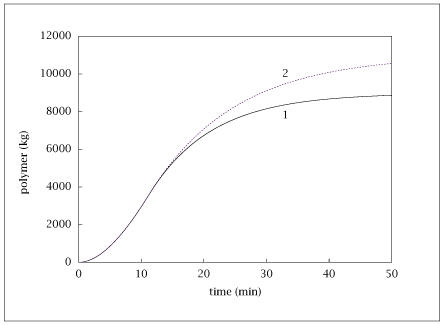## Figure 4.18:

### Semi-batch reactor polymer content for different monomer addition policies.## Code for Figure 4.18

### main.m

```
1
2
3
4
5
6
7
8
9
10
11
12
13
14
15
16
17
18
19
20
21
22
23
24
25
26
27
28
29
30
31
32
33
34
35
36
37
38
39
40
41
42
43
44
45
46
47
48
49
50
51
52
53
54
55
56
57
58
59
60
61
62
63
64
65
66
67
68
69
70
71
72
73
74
75
76
77
78
79
80
81
82
83
84
85
86
87
88
89
90
91
92
93
94
95
96
97
98
99
100
101
102
103
104
105
106
107
108
109
110
111
112
113% Copyright (C) 2001, James B. Rawlings and John G. Ekerdt
%
% This program is free software; you can redistribute it and/or
% modify it under the terms of the GNU General Public License as
% published by the Free Software Foundation; either version 2, or (at
% your option) any later version.
%
% This program is distributed in the hope that it will be useful, but
% WITHOUT ANY WARRANTY; without even the implied warranty of
% MERCHANTABILITY or FITNESS FOR A PARTICULAR PURPOSE.  See the GNU
% General Public License for more details.
%
% You should have received a copy of the GNU General Public License
% along with this program; see the file COPYING.  If not, write to
% the Free Software Foundation, 59 Temple Place - Suite 330, Boston,
% MA 02111-1307, USA.

% filling.m, semi-batch reactor polymerization with volume change.
% 1/17/00, jbr
%
% physical constants
%

% Revised 7/24/2018

k    = 0.1;   % min^-1
rhos = 0.9e3; % kg/m^3
rhom = 0.8e3; % kg/m^3
rhop = 1.1e3; % kg/m^3
% rhos = 1.0e3 % kg/m^3, not needed for calculations
% rhom = 1.0e3 % kg/m^3
% rhop = 1.0e3 % kg/m^3
VR   = 20;    % m^3
Qf0  = 1;     % m^3/min
Mm   = 100;   % kg/kmol
CM0  = 0;     % kmol/m^3
V0   = 10;    % m^3
%
% derived constants
%
CMf = rhom/Mm; % kmol/m^3
a   = Qf0*CMf; % kmol/min
deltaV = (1/rhop - 1/rhom)*Mm; % m^3/kmol
%
% find  filling time, tstop
%

p = struct(); %Strcture to pass parameters into fsolve function
p.k      = k;      % min^-1
p.VR     = VR;     % m^3
p.Qf0    = Qf0;    % m^3/min
p.V0     = V0;     % m^3
p.CMf    = CMf;    % kmol/m^3
p.deltaV = deltaV; % m^3/kmol
x0 = (VR - V0)/Qf0;
[x, fval, info] = fsolve(@(time) stopping(time,p),x0);
info;
tstop = x;
%
% plot solution
%
tfin = 50;
npts = tfin+1;
t1 = linspace(0,tstop,npts)';
V1 = V0 + (Qf0 + deltaV*Qf0*CMf)*t1 - deltaV*Qf0*CMf/k*(1-exp(-k*t1));
M1 = Qf0*CMf/k*(1-exp(-k*t1));
P1 = Qf0*CMf*( t1 - 1/k*(1-exp(-k*t1)) )*Mm;
t2 = linspace(tstop,tfin,npts)';
Qf1 = Qf0*ones(npts,1);
%
% Qf=0 for rest of operation
%
V20 = V1(npts);
M20 = M1(npts);
P20 = P1(npts);
M2  = M20*exp(-k*(t2-tstop));
P2  = P20 + M20*( 1-exp(-k*(t2-tstop)) )*Mm;
V2  = V20 + deltaV*M20*(1-exp(-k*(t2-tstop)));
Qf2 = zeros(npts,1);
%
% reactor is full for rest of operation
%
V3  = VR*ones(npts,1);
M3  = M20*exp( -k*(deltaV*CMf+1)*(t2-tstop) );
Qf3 = - deltaV*k*M3;
P3  = P20 + M20*k/(k*(deltaV*CMf+1))* ...
(1-exp( -k*(deltaV*CMf+1)*(t2-tstop) ))*Mm;
%
%save results for plotting
%
table = [ [t1;t2] [V1; V2] [M1; M2]*Mm [P1; P2] [Qf1; Qf2] ...
[V1; V3] [M1; M3]*Mm [P1; P3] [Qf1; Qf3] ];
save -ascii filling.dat table;

if (~ strcmp (getenv ('OMIT_PLOTS'), 'true')) % PLOTTING
subplot (2, 2, 1);
plot (table(:,1), [table(:,2),table(:,6)]);
axis ([0,50,-1,21])
% TITLE fillingV

subplot (2, 2, 2);
plot (table(:,1), [table(:,5),table(:,9)]);
axis ([0,50,-0.1,1.1]);
% TITLE fillingQ

subplot (2, 2, 3);
plot (table(:,1), [table(:,3),table(:,7)]);
% TITLE fillingM

subplot (2, 2, 4)
plot (table(:,1), [table(:,4),table(:,8)]);
% TITLE fillingP
end % PLOTTING

```

### stopping.m

```
1
2
3function retval = stopping (time,p)
retval = (p.VR - p.V0) - (p.Qf0 + p.deltaV*p.Qf0*p.CMf)*time...
+ p.deltaV*p.Qf0*p.CMf/p.k*(1 - exp(-p.k*time));

```